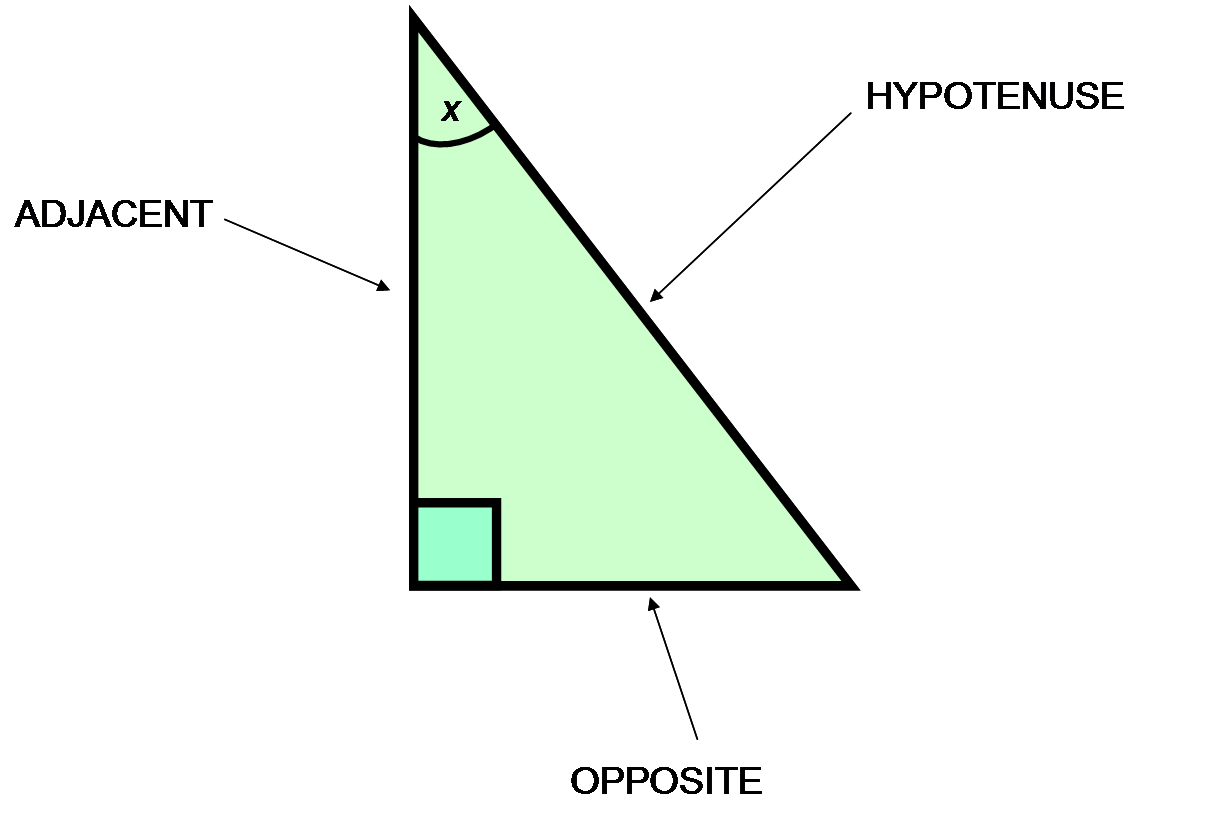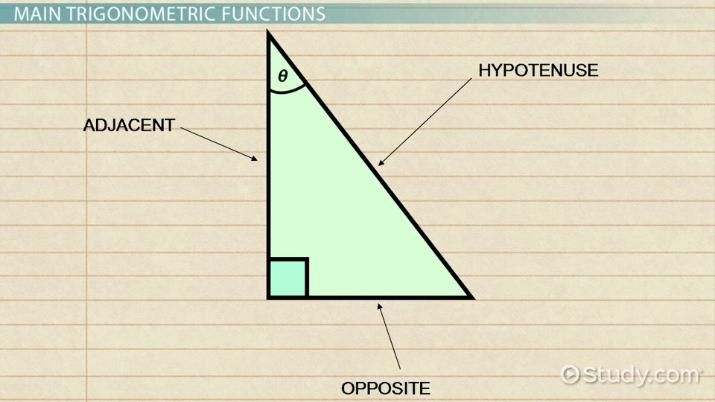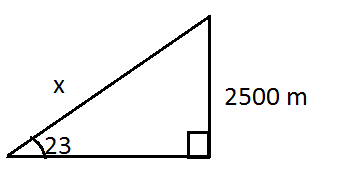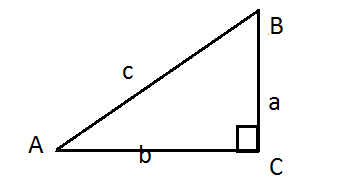Trigonometric Functions: Definition & Examples

Lesson Transcript
Instructor: Jennifer Beddoe

Jennifer has an MS in Chemistry and a BS in Biological Sciences.

There are six main trigonometric functions. Understand the definition and the main trigonometric functions, explore real-world examples, and how to solve problems. Updated: 09/09/2021

Main Trigonometric Functions

There are six main trigonometric functions:

• Sine (sin)
• Cosine (cos)
• Tangent (tan)
• Secant (sec)
• Cosecant (csc)
• Cotangent (cot)

These functions are used to relate the angles of a triangle with the sides of that triangle. Trigonometric functions are important when studying triangles and modeling periodic phenomena such as waves, sound, and light.

To define these functions for the angle theta, begin with a right triangle. Each function relates the angle to two sides of a right triangle. First, let's define the sides of the triangle.• The hypotenuse is the side opposite the right angle. The hypotenuse is always the longest side of a right triangle.
• The opposite side is the side opposite to the angle we are interested in, theta.
• The adjacent side is the side having both the angles of interest (angle theta and the right angle).

The relationship between the trigonometric functions and the sides of the triangle are as follows:

• sine(theta) = opposite / hypotenuse
• cosecant(theta) = hypotenuse / opposite
• cosine(theta) = adjacent / hypotenuse
• secant(theta) = hypotenuse / adjacent
• tangent(theta) = opposite / adjacent
• cotangent(theta) = adjacent / oppositeAn error occurred trying to load this video.

Try refreshing the page, or contact customer support.

Coming up next: Disk Method in Calculus: Formula & Examples

You're on a roll. Keep up the good work!

Replay
Your next lesson will play in 10 seconds
• 0:05 Main Trigonometric Functions
• 1:32 Real-World Examples
• 2:15 How to Solve Problems
• 4:07 Lesson Summary
Save Save

Want to watch this again later?

Timeline
Autoplay
Autoplay
Speed Speed

Real-World Examples

These trigonometric functions have practical applications in surveying, building, engineering, and even medicine. Here's one practical way to use these functions to solve a problem:The angle of elevation of an airplane is 23 degrees, and its altitude is 2500 meters. How far away is it?

We are trying to solve this right triangle for the hypotenuse x. Since the side length we know is opposite the angle we know, we can use the sine function.

Sin(23) = 2500m / x

x = 6398.3 meters

How to Solve Problems

You can use these ratios to solve for any side or angle of a right triangle. The information you are given will help you determine which function to use.

Example:Solve for b if you know that c is 2.5 km and B is 15.7 degrees.

To unlock this lesson you must be a Study.com Member.

Register to view this lesson

Are you a student or a teacher?